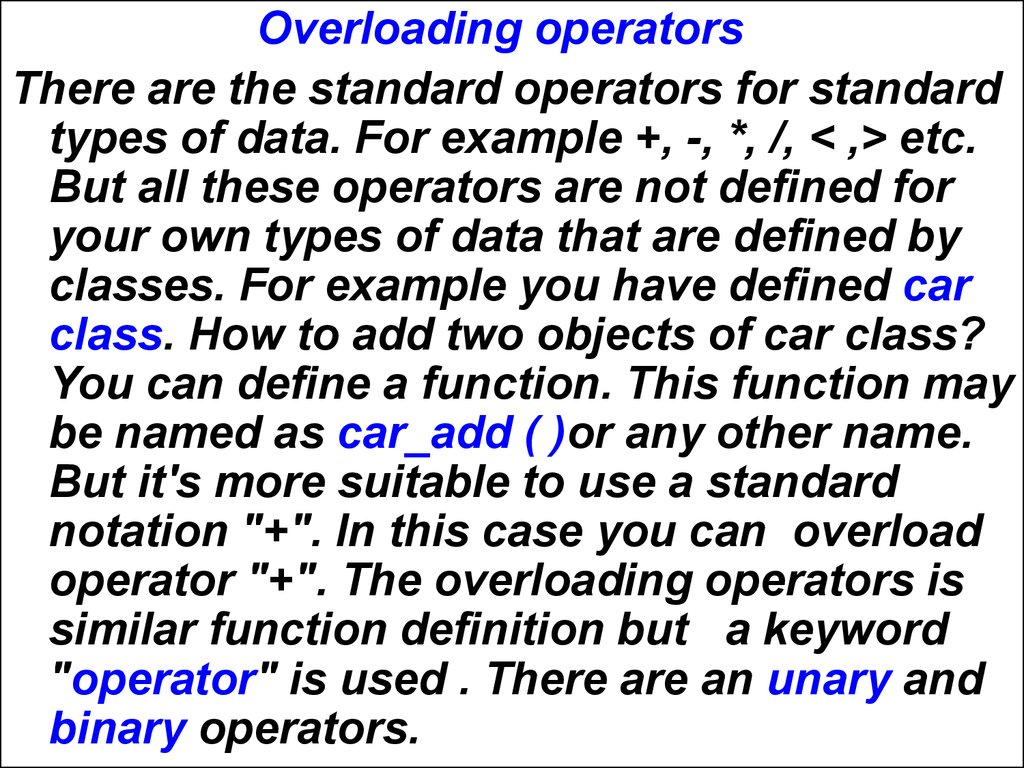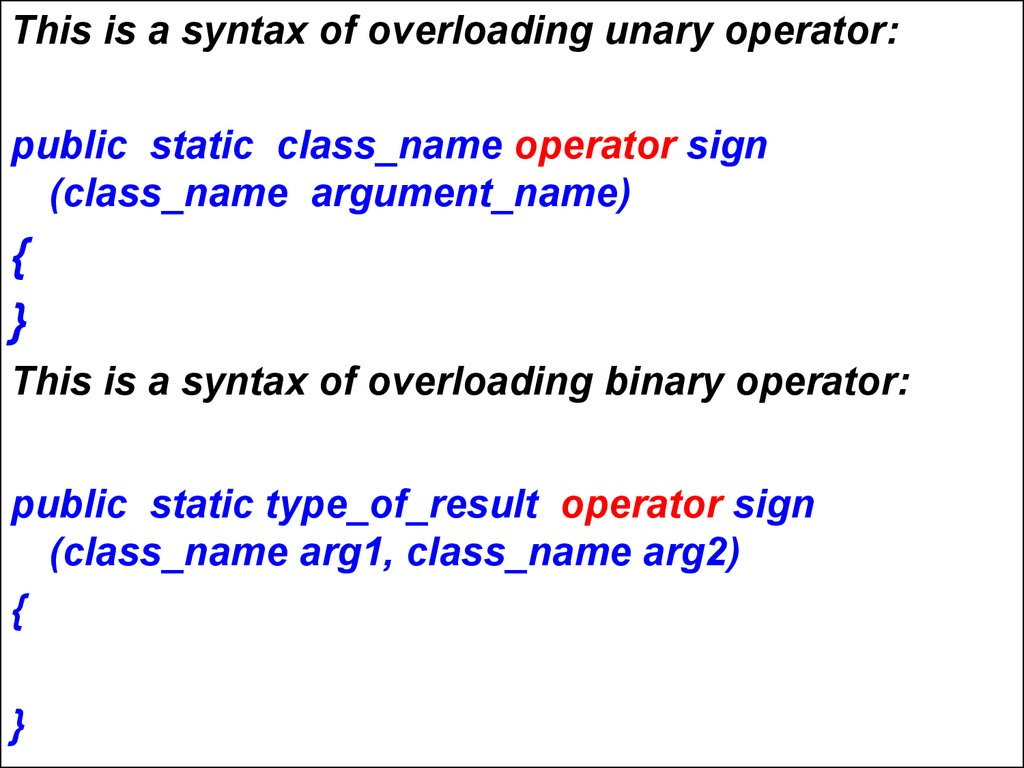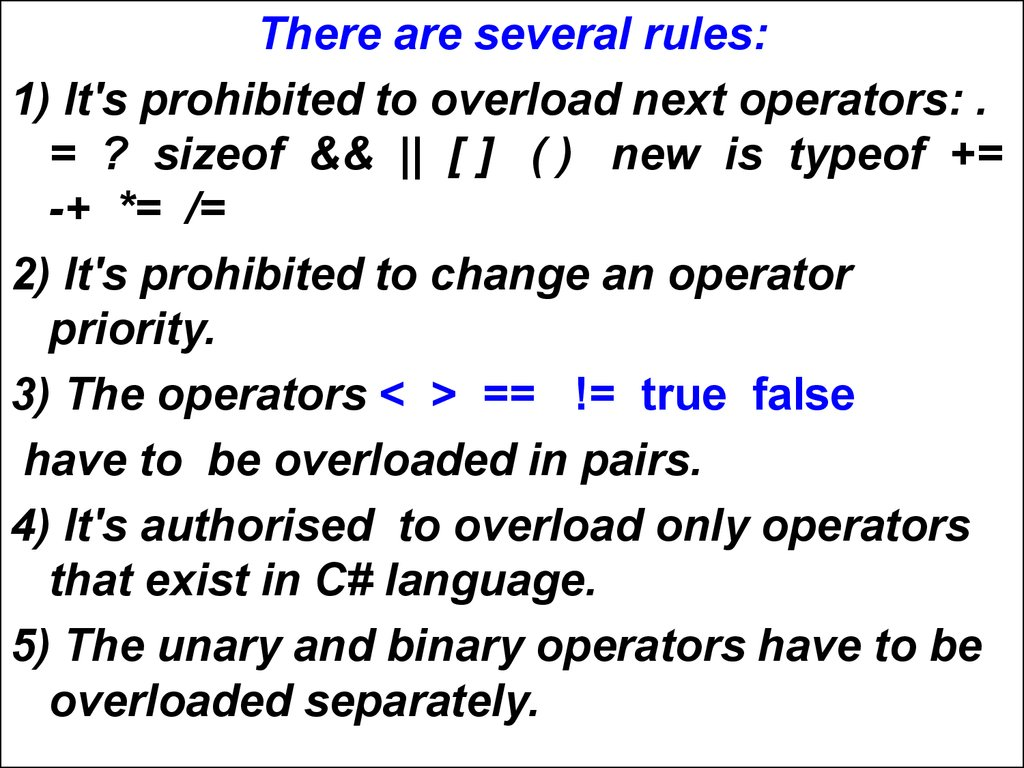# Lecture 8. Overloading operators c#

## 1.

Overloading operators
There are the standard operators for standard
types of data. For example +, -, *, /, < ,> etc.
But all these operators are not defined for
your own types of data that are defined by
classes. For example you have defined car
class. How to add two objects of car class?
You can define a function. This function may
be named as car_add ( )or any other name.
But it's more suitable to use a standard
notation "+". In this case you can overload
operator "+". The overloading operators is
similar function definition but a keyword
"operator" is used . There are an unary and
binary operators.

## 2.

This is a syntax of overloading unary operator:
public static class_name operator sign
(class_name argument_name)
{
}
This is a syntax of overloading binary operator:
public static type_of_result operator sign
(class_name arg1, class_name arg2)
{
}

## 3.

There are several rules:
1) It's prohibited to overload next operators: .
= ? sizeof && || [ ] ( ) new is typeof +=
-+ *= /=
2) It's prohibited to change an operator
priority.
3) The operators < > == != true false
have to be overloaded in pairs.
4) It's authorised to overload only operators
that exist in C# language.
5) The unary and binary operators have to be
overloaded separately.

## 4.

There are several examples:
public static bool operator ==(car x, car y)
{
if (x.brand == y.brand && x.max_speed
== y.max_speed && x.amount_of_passenger
== y.amount_of_passenger)
return true;
else
return false;
}

## 5.

public static bool operator !=(car x, car y)
{
if (x.brand != y.brand || x.max_speed !=
y.max_speed || x.amount_of_passenger !=
y.amount_of_passenger )
return true;
else
return false;
}

## 6.

public static int operator+(car x, car y)
{
return x.amount_of_passenger +
y.amount_of_passenger;
}

## 7.

Task
It's necessary to create a class of complex
numbers with next member variables:
real part and imagine part. Must be
input() and output() member functions
also. Besides of it's necessary to
overload next operators: "+", unary "-",
"==", "!="

## 8.

using System;
using System.Collections.Generic;
using System.Text;
namespace overcomp1
{
class comp
{
double Re, Im;
public void input(string nch)
{
string s;
Console.WriteLine("Enter {0}.Re=",nch);
s = Console.ReadLine();
Re = Convert.ToDouble(s);
Console.WriteLine("Enter {0}.Im=",nch);
s = Console.ReadLine();
Im = Convert.ToDouble(s);
}

## 9.

public void output(string nch)
{
Console.WriteLine("{0}.Re={1} {2}.Im={3}",nch, Re, nch,Im);
}
public static comp operator +(comp a, comp b)
{
comp v = new comp();
v.Re = a.Re + b.Re;
v.Im = a.Im + b.Im;
return v;
}
public static comp operator-(comp a)
{
a.Re=-a.Re;
a.Im=-a.Im;
return a;
}

## 10.

public static bool operator==(comp a, comp b)
{
if (a.Re == b.Re && a.Im == b.Im)
return true;
else
return false;
}
public static bool operator !=(comp a, comp b)
{
if( a.Re != b.Re ||a.Im != b.Im)
return true;
else
return false;
}
}

## 11.

class Program
{
static void Main(string[] args)
{ comp c1=new comp();
comp c2=new comp();
comp c3=new comp();
c1.input("c1");
c2.input("c2");
c3 = c1 + c2;
c3.output("c3");
c3=-c1;
c3.output("c3");
if (c2 == c1)
Console.WriteLine("c1 == c2");
else
Console.WriteLine("c1 != c2");
}
}
}

## 12.

The example
It's necessary to overload next
comparison operators for Flower class:
< , >. There are next member variables
of Flower class: name, color, height,
price. You must solve independently
which member variables are used for
comparing.

## 13.

using System;
using System.Collections.Generic;
using System.Text;
namespace flower
{
class flower
{
string name;
string color;
double height;
double price;
public void input()
{
string s;
Console.WriteLine("Enter name");
name = Console.ReadLine();
Console.WriteLine("Enter color");
color = Console.ReadLine();
Console.WriteLine("Enter height");
s = Console.ReadLine();

## 14.

height = Convert.ToDouble(s);
Console.WriteLine("Enter price");
s = Console.ReadLine();
price = Convert.ToDouble(s);
}
public static bool operator <(flower a, flower b)
{
if (a.price < b.price)
return true;
else
return false;
}
public static bool operator >(flower a, flower b)
{
if (a.price > b.price)
return true;
else
return false;
}
}

## 15.

class Program
{
static void Main(string[] args)
{
flower x = new flower();
x.input();
flower y = new flower();
y.input();
bool result;
result = x < y;
Console.WriteLine("result={0}",result);
}
}
}

## 16.

Now do the next program:
Task
• To overload operation for addition of two
vectors. This operation signed as +.